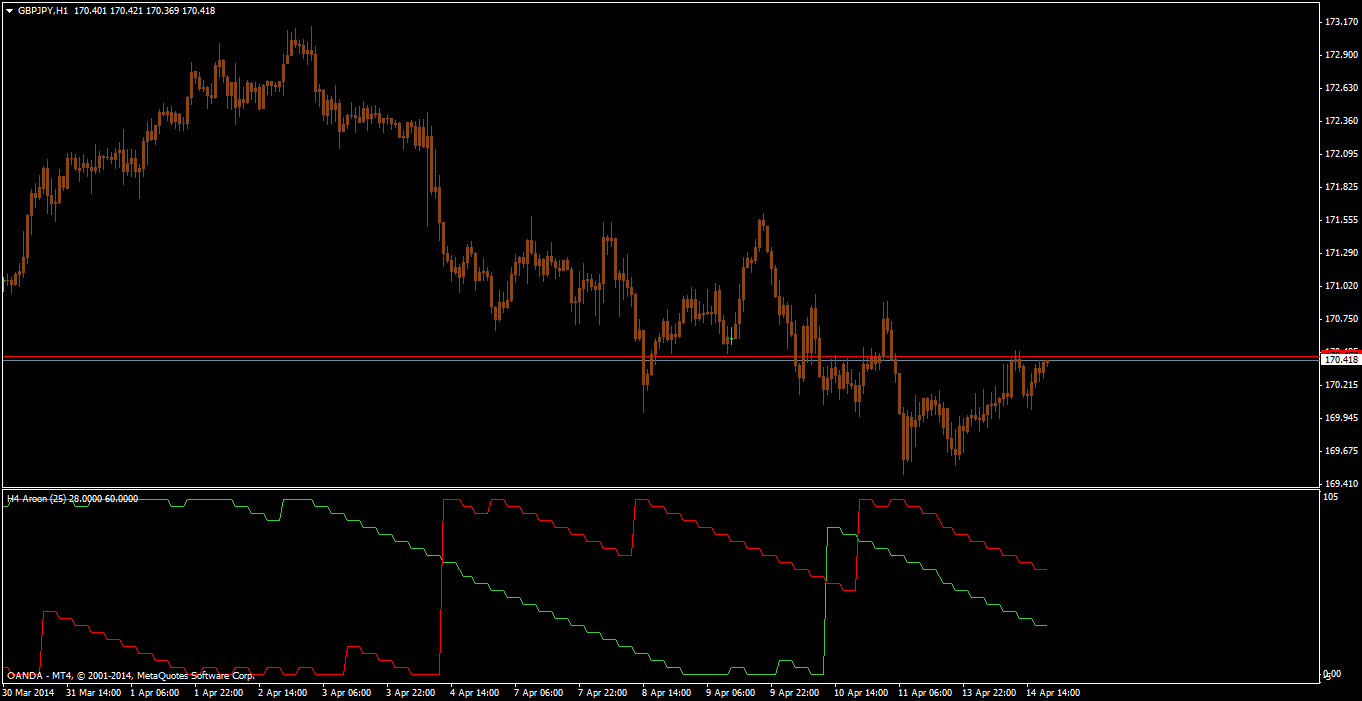# Indicators with alerts/signal - page 134260

mladen or anyone, i found an indicator in forex tsd but i lost it., mladen i think you modified it. it was a mtf moving average indicator with alerts. it had a drop down box for type of ma and a drop down box for ma period. any help appreciated.9552

Aroon & alerts mtf from here: https://www.mql5.com/en/forum/180648/page418 made to be compatible with new mt4 builds.Files:244

craig65:
mladen or anyone, i found an indicator in forex tsd but i lost it., mladen i think you modified it. it was a mtf moving average indicator with alerts. it had a drop down box for type of ma and a drop down box for ma period. any help appreciated.

Maybe this one? It has got mtf, alerts and 30 MA calculations to chose from.

allaveragescrossover_v1.8_600.mq4

Files:207278

craig65:
mladen or anyone, i found an indicator in forex tsd but i lost it., mladen i think you modified it. it was a mtf moving average indicator with alerts. it had a drop down box for type of ma and a drop down box for ma period. any help appreciated.

craig65

You can try out the version posted by rplust or you can try out this averages (they are different in may ways) : https://www.mql5.com/en/forum/general

____________________

In either case, the drop down box for ma periods is not available (periods are usually not entered that way)[Deleted]

I have a request to you

Can you add 5 period TMAcentered (median) indicator to this stepma_line mq5 file?

If you do I will be very pleased.

Regards

suat

Files:237

Hello , i'm looking to add alert to an indicator , help not forthcoming but still trying . I understand i have to find the SETINDEXBUFFER , in this case , the code of the indicator has 4 setindexbuffers , if i'm not wrong , now i am unable to find the buffer setting value in the indicator where i presumably have to add the following code ''Alert("Indicator set");}''to every buffer setting thereafter ...

Could anyone kind enough assist me in doing this ? I' seeing code for the first time in my life and it's hard to understand . Here is the code of the indicator

void init() {

IndicatorDigits(2);

SetIndexBuffer(0, g_ibuf_88);

SetIndexLabel(0, "LineaSqCD");

SetIndexStyle(0, DRAW_LINE, STYLE_SOLID, 3);

SetIndexBuffer(1, g_ibuf_92);

SetIndexLabel(1, "LineaSqCD");

SetIndexStyle(1, DRAW_LINE, STYLE_SOLID, 3);

SetIndexBuffer(2, g_ibuf_96);

SetIndexLabel(2, "LineaSqCD");

SetIndexStyle(2, DRAW_NONE);

SetIndexBuffer(3, g_ibuf_100);

SetIndexLabel(3, "LineaSqCD");

SetIndexStyle(3, DRAW_NONE);

Comment("");

}

int start() {

double ld_0;

double ld_8;

double ld_16;

double ld_24;

int li_44;

int li_48;

double l_ima_52;

int li_40 = IndicatorCounted();

if (li_40 >= 0) {

if (li_40 > 0) li_40--;

li_40 = Bars + 1 - li_40;

for (int li_36 = 0; li_36 < li_40; li_36++) {

g_ibuf_92[li_36] = -gi_84;

g_ibuf_88[li_36] = -gi_84;

l_ima_52 = iMA(NULL, 0, g_period_76, 0, MODE_EMA, PRICE_TYPICAL, li_36);

if (Close[li_36] > l_ima_52) g_ibuf_92[li_36] = EMPTY_VALUE;

if (Close[li_36] < l_ima_52) g_ibuf_88[li_36] = EMPTY_VALUE;

}

li_44 = Bars + 1 - gi_80 - 5;

li_48 = li_44 - gi_80 - 1;

for (li_36 = li_48; li_36 >= 0; li_36--) {

ld_0 = 0;

for (int li_32 = gi_80; li_32 >= 1; li_32--) {

ld_8 = gi_80 + 1;

ld_8 /= 3.0;

ld_16 = 0;

ld_16 = (li_32 - ld_8) * (Close[gi_80 - li_32 + li_36]);

ld_0 += ld_16;

}

ld_24 = 6.0 * ld_0 / (gi_80 * (gi_80 + 1));

g_ibuf_96[li_36] = gi_84;

g_ibuf_100[li_36] = gi_84;

if (ld_24 > Close[li_36]) g_ibuf_100[li_36] = EMPTY_VALUE;

if (ld_24 < Close[li_36]) g_ibuf_96[li_36] = EMPTY_VALUE;

}

}

return (0);

}2973

Stan007:
Hello , i'm looking to add alert to an indicator , help not forthcoming but still trying . I understand i have to find the SETINDEXBUFFER , in this case , the code of the indicator has 4 setindexbuffers , if i'm not wrong , now i am unable to find the buffer setting value in the indicator where i presumably have to add the following code ''Alert("Indicator set");}''to every buffer setting thereafter ...

Could anyone kind enough assist me in doing this ? I' seeing code for the first time in my life and it's hard to understand . Here is the code of the indicator

void init() {

IndicatorDigits(2);

SetIndexBuffer(0, g_ibuf_88);

SetIndexLabel(0, "LineaSqCD");

SetIndexStyle(0, DRAW_LINE, STYLE_SOLID, 3);

SetIndexBuffer(1, g_ibuf_92);

SetIndexLabel(1, "LineaSqCD");

SetIndexStyle(1, DRAW_LINE, STYLE_SOLID, 3);

SetIndexBuffer(2, g_ibuf_96);

SetIndexLabel(2, "LineaSqCD");

SetIndexStyle(2, DRAW_NONE);

SetIndexBuffer(3, g_ibuf_100);

SetIndexLabel(3, "LineaSqCD");

SetIndexStyle(3, DRAW_NONE);

Comment("");

}

int start() {

double ld_0;

double ld_8;

double ld_16;

double ld_24;

int li_44;

int li_48;

double l_ima_52;

int li_40 = IndicatorCounted();

if (li_40 >= 0) {

if (li_40 > 0) li_40--;

li_40 = Bars + 1 - li_40;

for (int li_36 = 0; li_36 < li_40; li_36++) {

g_ibuf_92[li_36] = -gi_84;

g_ibuf_88[li_36] = -gi_84;

l_ima_52 = iMA(NULL, 0, g_period_76, 0, MODE_EMA, PRICE_TYPICAL, li_36);

if (Close[li_36] > l_ima_52) g_ibuf_92[li_36] = EMPTY_VALUE;

if (Close[li_36] < l_ima_52) g_ibuf_88[li_36] = EMPTY_VALUE;

}

li_44 = Bars + 1 - gi_80 - 5;

li_48 = li_44 - gi_80 - 1;

for (li_36 = li_48; li_36 >= 0; li_36--) {

ld_0 = 0;

for (int li_32 = gi_80; li_32 >= 1; li_32--) {

ld_8 = gi_80 + 1;

ld_8 /= 3.0;

ld_16 = 0;

ld_16 = (li_32 - ld_8) * (Close[gi_80 - li_32 + li_36]);

ld_0 += ld_16;

}

ld_24 = 6.0 * ld_0 / (gi_80 * (gi_80 + 1));

g_ibuf_96[li_36] = gi_84;

g_ibuf_100[li_36] = gi_84;

if (ld_24 > Close[li_36]) g_ibuf_100[li_36] = EMPTY_VALUE;

if (ld_24 < Close[li_36]) g_ibuf_96[li_36] = EMPTY_VALUE;

}

}

return (0);

}

That is decompiled code237

Hi techmac , I actually didn't know it was decompiled , just found the thing on the internet . Being decompiled makes it impossible to code an alert into it ?207278

Stan007:
Hi techmac , I actually didn't know it was decompiled , just found the thing on the internet . Being decompiled makes it impossible to code an alert into it ?

Stan007

Being decompiled will make a lot of coders refuse to work on it (since decompiled code is a stolen code in 99.99% of cases)4411